Homework Help Question & Answers

# s points) Given the two points P2,4) and Qu,-5) (a) Find the distance between P and...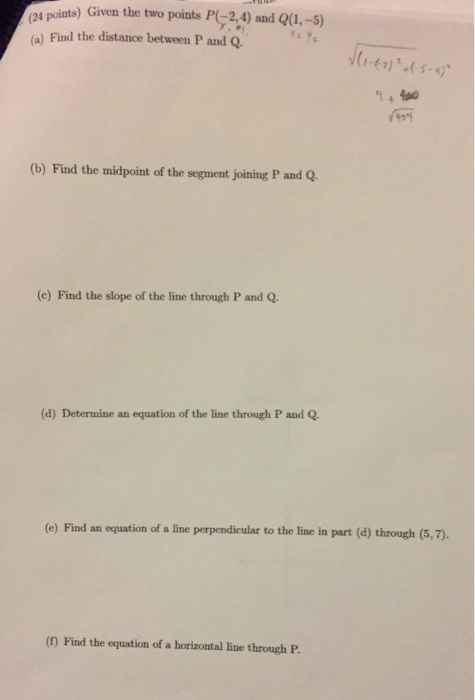s points) Given the two points P2,4) and Qu,-5) (a) Find the distance between P and Q (b) Find the midpoint of the segment joining P and Q. (c) Find the slope of the line through P and Q. (d) Determine an equation of the line through P and Q (e) Find an equation of a line perpendicular to the line in part (d) through (5,7). (f) Find the equation of a horizontal line through P.

#### Homework Answers

Answer #1

P = ( - 2 , 4 ) , Q = ( 1, - 5)

a) distance = sqrt [ ( - 5 - 4)^2 + ( 1 + 2)^2 ]

distance = sqrt 90

distance = 3 sqrt 10

b) mid point = ( - 2 + 1 / 2 , 4 + 5 / 2 )

= (-1/2 , 9/2 )

c) slope = y2 - y2 / x2 - x1

= ( - 5 - 4 ) / ( 1 + 2 )

slope = - 3

d) equation of line

y - y1 = m ( x- x1 )

y - 4 = - 3 ( x+ 2 )

y = - 3x - 2

e) equation of perpendicular line

slope of perpendicular line = 1/3

y - 7 = 1/3 ( x- 5)

y = 1/3 x + 16/3

f) equation of horizontal line through P

y = 4

Know the answer?
Your Answer:

#### Post as a guest

Your Name:

What's your source?

#### Earn Coin

Coins can be redeemed for fabulous gifts.

Not the answer you're looking for? Ask your own homework help question. Our experts will answer your question WITHIN MINUTES for Free.
Similar Homework Help Questions
• ### Let S denote the sphere x2 y2 2 = 1. Given two points P(1,0,0), (a) Find the distance between P and Q. Lets call this E...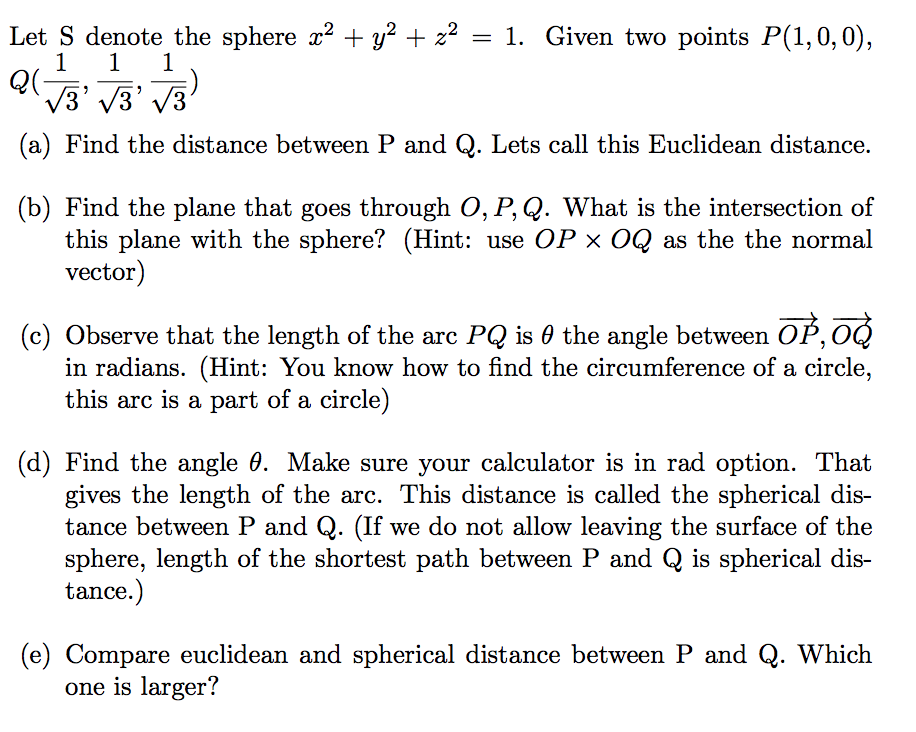Let S denote the sphere x2 y2 2 = 1. Given two points P(1,0,0), (a) Find the distance between P and Q. Lets call this Euclidean distance. (b) Find the plane that goes through O, P, Q. What is the intersection of this plane with the sphere? (Hint: use OP × OQ as the the normal vector) (c) Observe that the length of the arc PQ is 0 the angle between OP,0Q in radians. (Hint: You know how to find...

• ### (-6,-4),(3,4) FIND THE DISTANCE BETWEEN EACH PAIR OF POINTS AND THE MIDPOINT OF THE LINE SEGMENT JOINING THE POINTS

(-6,-4),(3,4) FIND THE DISTANCE BETWEEN EACH PAIR OF POINTS AND THE MIDPOINT OF THE LINE SEGMENT JOINING THE POINTS. LEAVE DISTANCE IN RADICAL FORM. THANKS

• ### Find the distance between (2, -4) and (1,-6). Give the exact distance. midpoint of the line...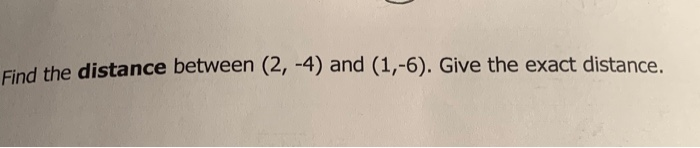Find the distance between (2, -4) and (1,-6). Give the exact distance. midpoint of the line segment that joins points P(2,3) and Q(-2,5).

• ### QUESTION 4 Use the distance formula to find the distance between the two points. 4-6, 19)...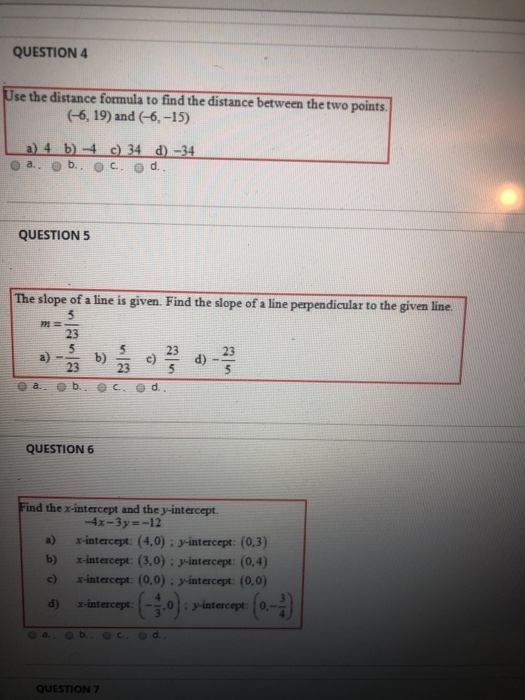QUESTION 4 Use the distance formula to find the distance between the two points. 4-6, 19) and (-6, -15) a) 4 b) 4 a.. b.. c) 34 . d) -34 d. QUESTION 5 The slope of a line is given. Find the slope of a line perpendicular to the given line. lu b.ec d QUESTION 6 Find the x-intercept and the y-intercept. 4x-3y=-12 a) x-intercept: (4,0) : y-intercept: (0,3) b) x-intercept: (3,0): y-intercept: (0,4) c) -intercept: (0,0): y-intercept: (0,0) a)...

• ### Two points P and Q are given. P(2, 1, 0), Q(−1, 2, −3) (a) Find the distance between P and Q.

Two points P and Q are given. P(2, 1, 0), Q(−1, 2, −3) (a) Find the distance between P and Q.

• ### 2) Determine the direction and magnitude of the electric field at the point P shown in...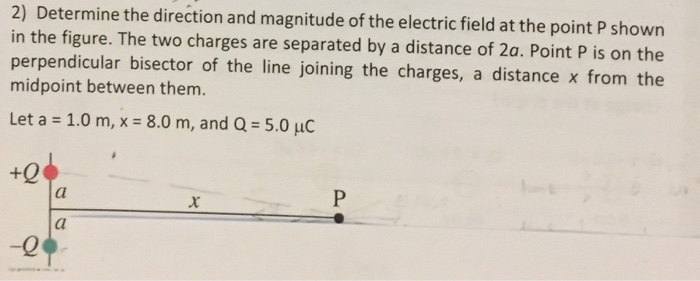2) Determine the direction and magnitude of the electric field at the point P shown in the figure. The two charges are separated by a distance of 2a. Point P is on the perpendicular bisector of the line joining the charges, a distance x from the midpoint between them. Let a = 1.0 m, x-8.0 m, and Q= 5.0 uc -0

• ### Determine magnitude of the electric field at the point P shown in the figure(Figure 1) ....

Determine magnitude of the electric field at the point P shown in the figure(Figure 1) . The two charges are separated by a distance of 2a. Point P is on the perpendicular bisector of the line joining the charges, a distance x from the midpoint between them. Determine the direction of the electric field. Assume that the positive x and y axes are directed to the right and upward respectively. Determine magnitude of the electric field at the point P...

• ### Find the midpoint of the line segment joining the points P, and P2 P1 = (-0.3,1.2)...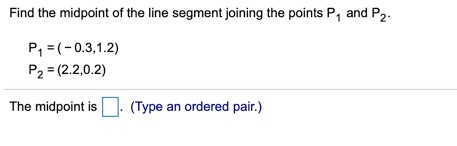Find the midpoint of the line segment joining the points P, and P2 P1 = (-0.3,1.2) P2 = (2-2,0.2) The midpoint is . (Type an ordered pair.)

• ### I need some help with this problem, please. Problem 1 Consider the points P = (01,02)...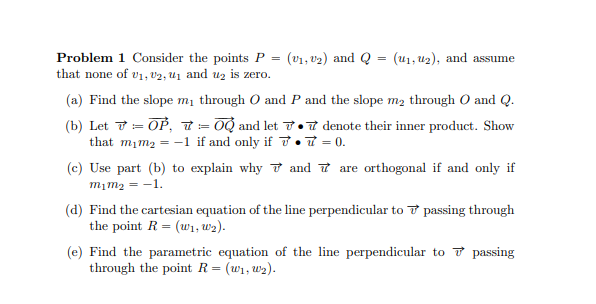I need some help with this problem, please. Problem 1 Consider the points P = (01,02) and Q = (U1, U2), and assume that none of 01, 02, u and uz is zero. (a) Find the slope my through O and P and the slope m2 through O and Q. (b) Let T O P, 7=and let 7.7 denote their inner product. Show that mim2 = -1 if and only if 7.7 = 0. (c) Use part (b) to explain...

• ### Please answer both questions. Determine magnitude of the electric field at the point P shown in...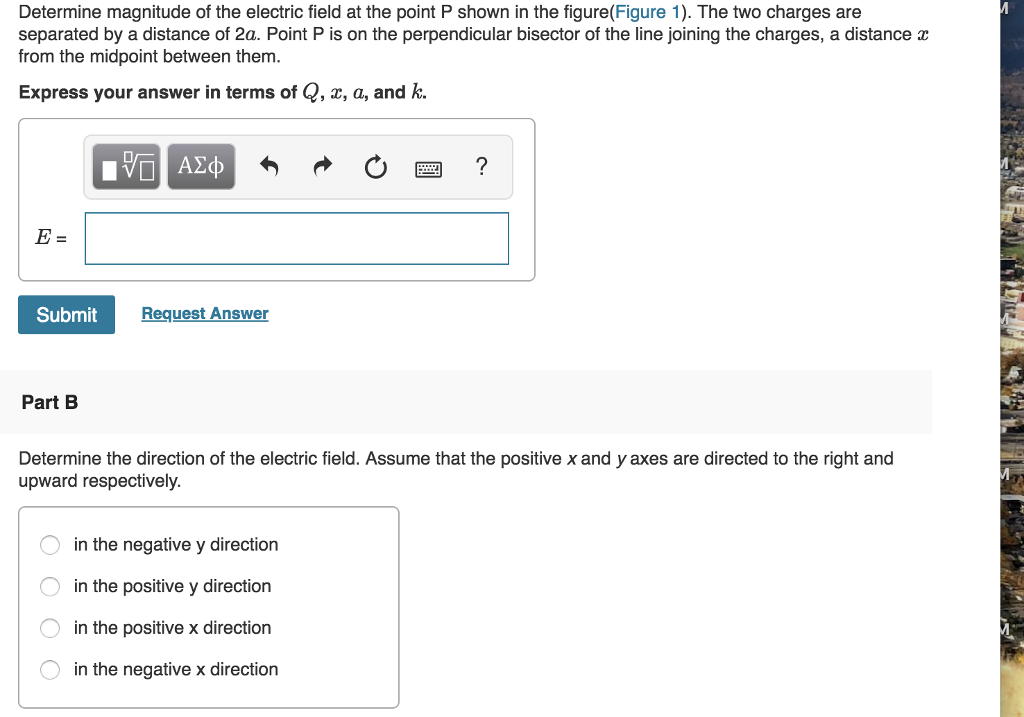Please answer both questions. Determine magnitude of the electric field at the point P shown in the figure(Figure 1). The two charges are separated by a distance of 2a. Point P is on the perpendicular bisector of the line joining the charges, a distance from the midpoint between them. Express your answer in terms of Q, z, a, and k. E= Submit Request Answer Part B Determine the direction of the electric field. Assume that the positive x and y...

Free Homework App

Scan Your Homework
to Get Instant Free Answers
Need Online Homework Help?

Get Answers For Free
Most questions answered within 3 hours.